# Ordered group

A groupwith an order relationsuch that for any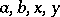inthe inequalityentails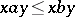. If the order is total (respectively, partial), one speaks of a totally ordered group (respectively, a partially ordered group).

An order homomorphism of a (partially) ordered groupinto an ordered groupis a homomorphismofintosuch that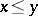,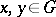, impliesin. The kernels of order homomorphisms are the convex normal subgroups (cf. Convex subgroup; Normal subgroup). The set of right cosets of a totally ordered groupwith respect to a convex subgroupis totally ordered by putting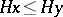if and only if. Ifis a convex normal subgroup of a totally ordered group, then this order relation turns the quotient group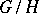into a totally ordered group.

The system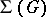of convex subgroups of a totally ordered group possesses the following properties: a)is totally ordered by inclusion and closed under intersections and unions; b)is infra-invariant, i.e. for anyand any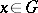one has; c) ifis a jump in, i.e.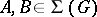,, and there is no convex subgroup between them, thenis normal in, the quotient groupis an Archimedean group and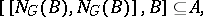where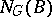is the normalizer ofin(cf. Normalizer of a subset); and d) all subgroups ofare strongly isolated, i.e. for any finite set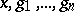inand any subgroupthe relation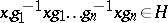entails.

An extensionof an ordered groupby an ordered group (cf. Extension of a group) is an ordered group if the order inis stable under all inner automorphisms of. An extensionof an ordered groupby a finite group is an ordered group ifis torsion-free and if the order inis stable under all inner automorphisms of.

The order type of a countable ordered group has the form, where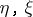are the order types of the set of integers and of rational numbers, respectively, andis an arbitrary countable ordinal. Every ordered groupis a topological group relative to the interval topology, in which a base of open sets consists of the open intervals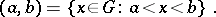A convex subgroup of an ordered group is open in this topology.